### 1. 前言

• 本文总结的内容如下
• 深度可分离卷积Depthwise separable convolution和标准卷积
• 两者params参数量和计算量的对比

### 2. Depthwise separable convolution

• 深度可分离卷积就是将普通卷积拆分成为一个深度卷积和一个逐点卷积
• 先来看一下标准的卷积操作：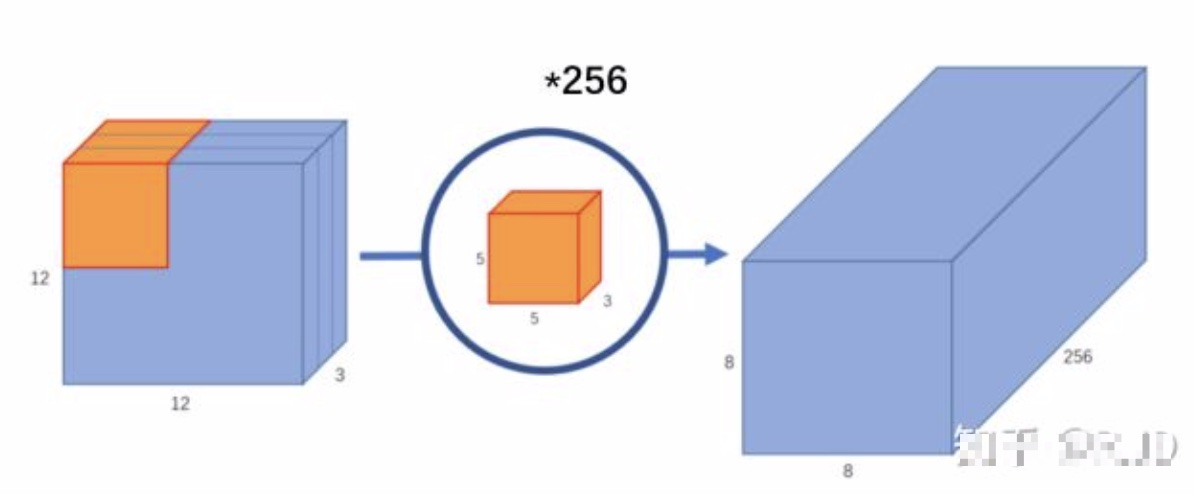• 输入一个12×12×3的一个输入特征图，经过5×5×3的卷积核卷积得到一个8×8×1的输出特征图。如果此时我们有256个卷积核，我们将会得到一个8×8×256的输出特征图。

• 普通卷积分离出的深度卷积如下：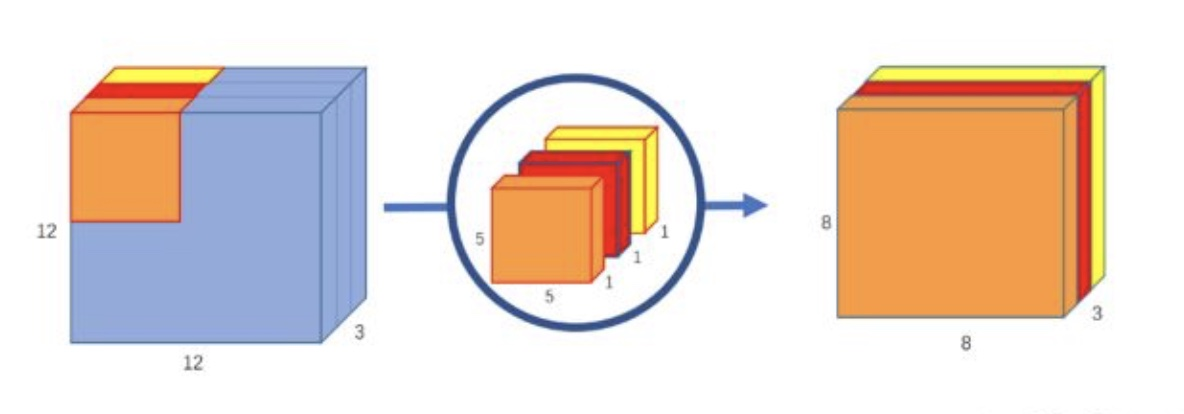• 与标准卷积网络不一样的是，我们将卷积核拆分成为但单通道形式，在不改变输入特征图像的深度的情况下，对每一通道进行卷积操作，这样就得到了和输入特征图通道数一致的输出特征图
• 如上图：输入12×12×3的特征图，经过5×5×1×3的深度卷积之后，得到了8×8×3的输出特征图。输入和输出的维度是不变的3。

• 分离的逐点卷积如下：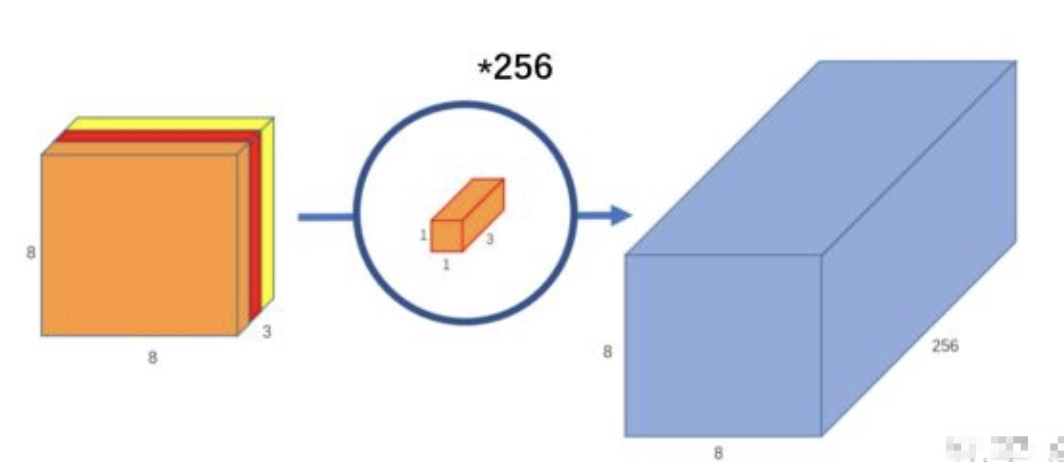• 在深度卷积的过程中，我们得到了8×8×3的输出特征图，我们用256个1×1×3的卷积核对输入特征图进行卷积操作，输出的特征图和标准的卷积操作一样都是8×8×256了。
• 标准卷积和深度可分离卷积的对比图示：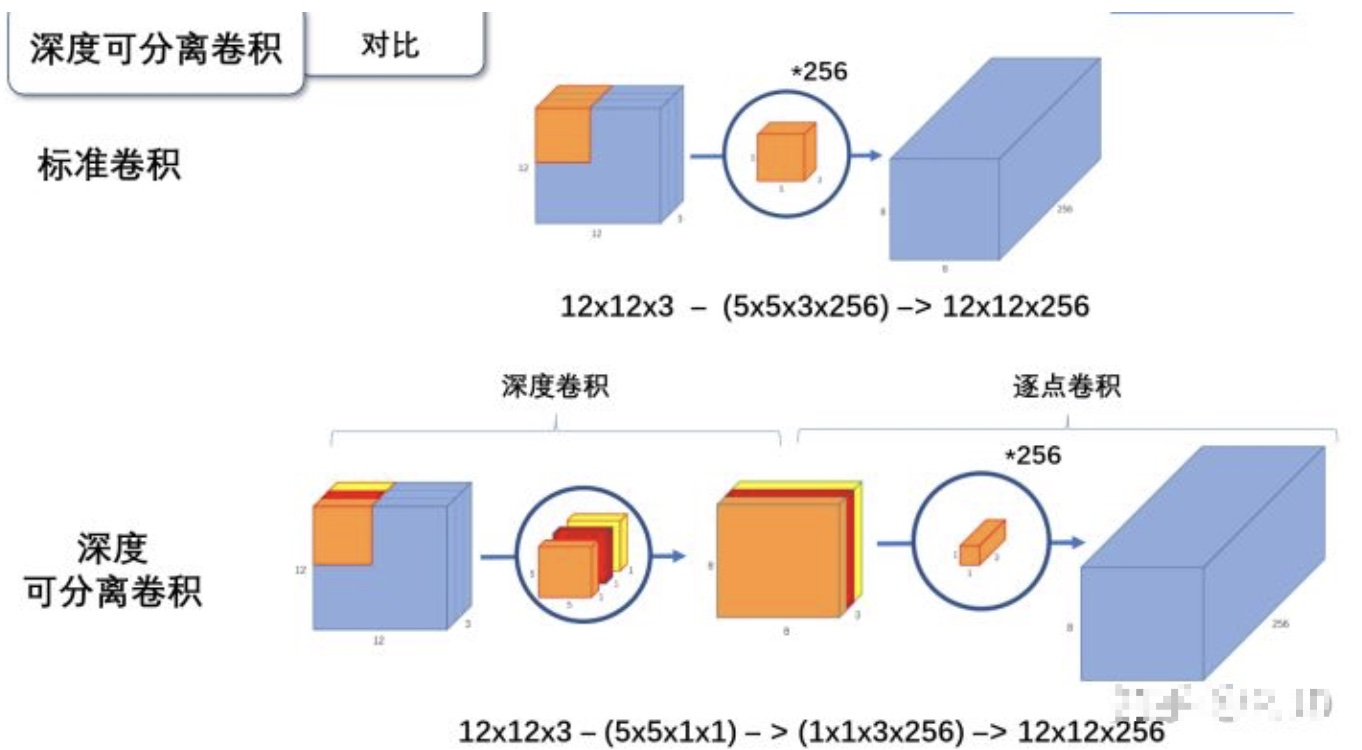### 3. 卷积参数量和计算量

• 计算标准卷积的参数量和计算量：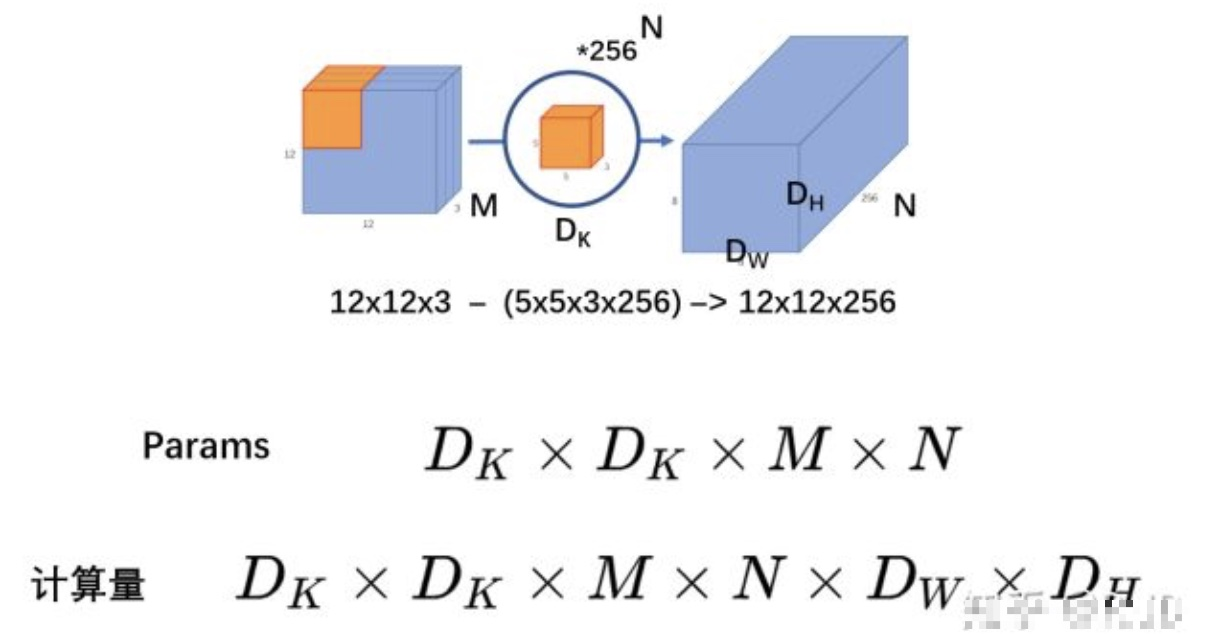• 计算参数量要看卷积核：卷积核的尺寸$D_k \times D_k \times M$，卷积核的个数$N$，所以标准卷积的参数量为：
• 计算量要考虑卷积核和输入的大小：卷积核的尺寸$D_k \times D_k \times M$，卷积核的个数$N$，每一个卷积核都要进行$D_w \times D_h$次操作，所以标准卷积的计算量为：
• 计算深度可分离卷积的参数量和计算量：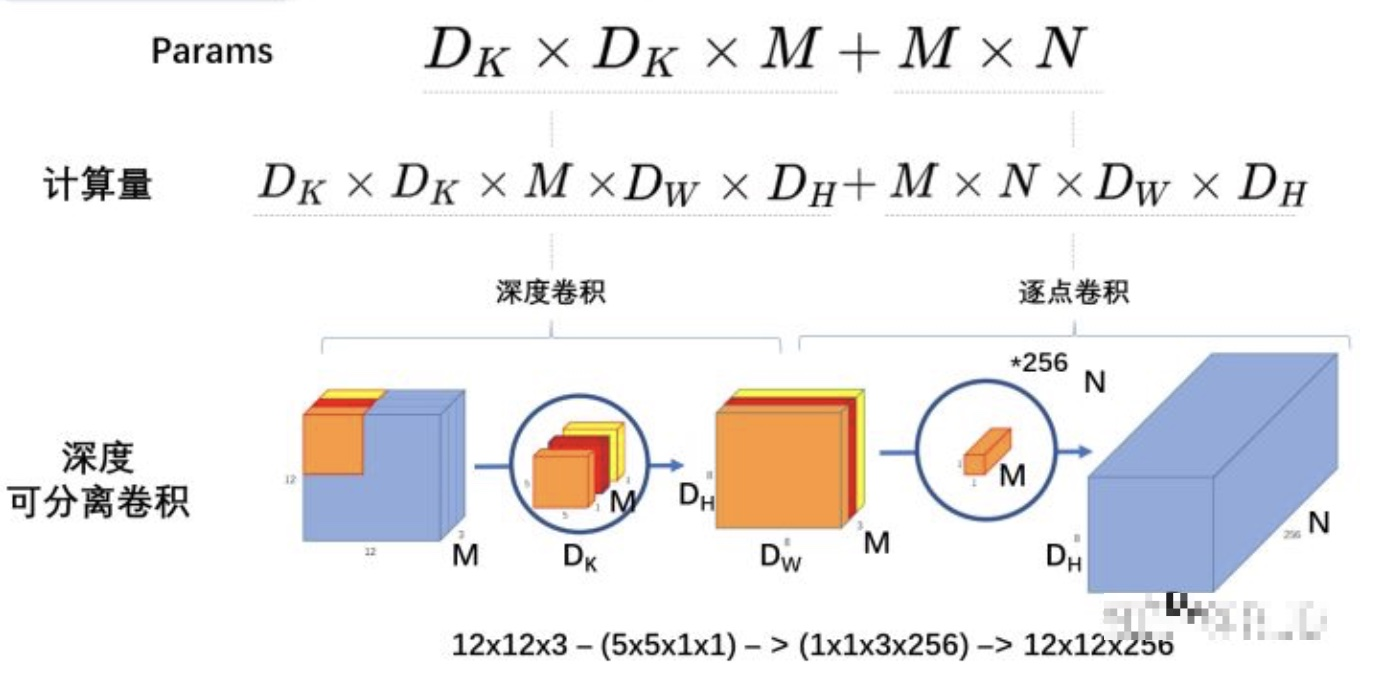• 计算参数量需要考虑整个过程的卷积核：深度卷积中$M$个$D_k \times D_k$大小的卷积核，参数量为$D_k \times D_k \times M$，逐点卷积中$N$个$1\times1\times M$大小的卷积核，参数量为$M \times N$，所以整个过程的参数量：
• 计算量的计算： 在上述参数量的基础上，每次做$D_w \times D_h$ 次计算，所以计算量：
• 对比标准卷积和深度分离卷积：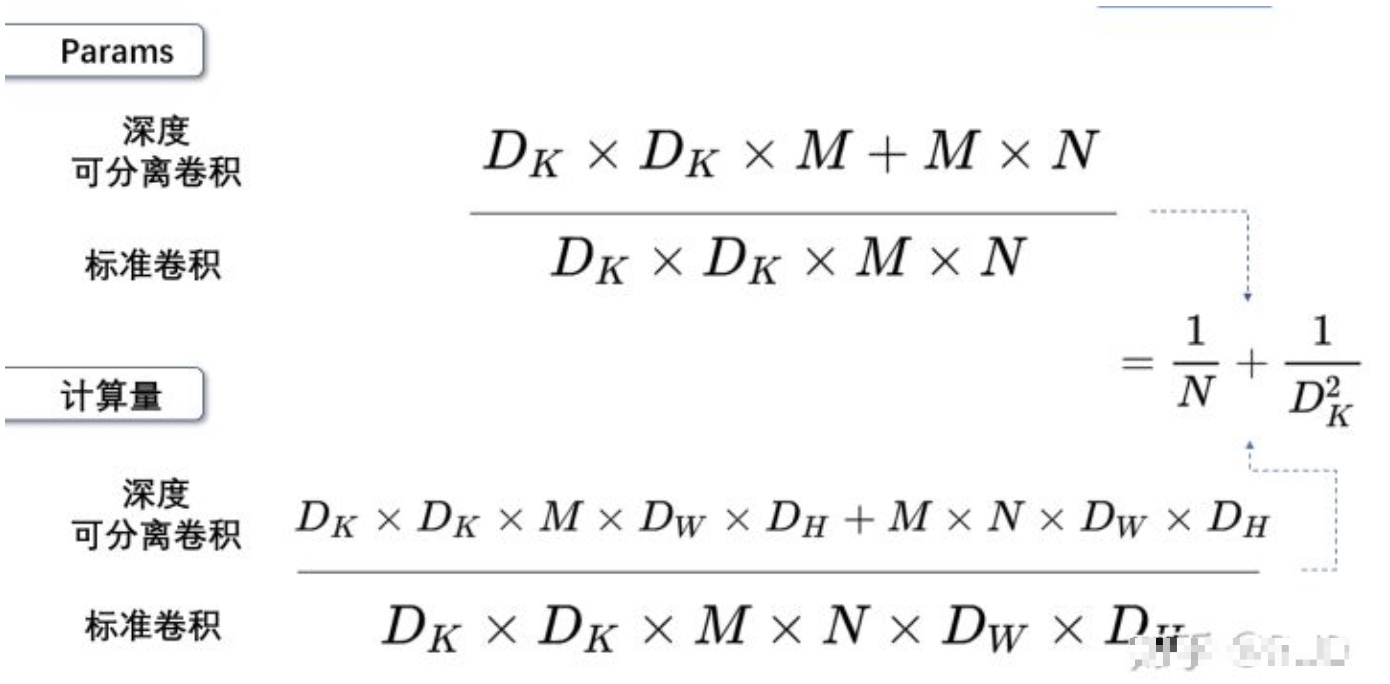• 由上图可知，深度分离卷积参数量和计算量都为标准卷积的$\frac{1}{N} + \frac{1}{D_k^2}$ ，我们通常所使用的是3×3的卷积核，也就是会下降到原来的九分之一到八分之一
-------------The End-------------